﻿ Gotta Fish Carburetors: Calculate Your Engine's CFM RequirementsGOTTA FISH CARBURETORS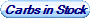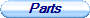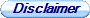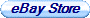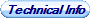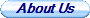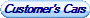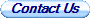All carburetors are rated in their ability to take in air in Cubic Feet per Minute (CFM). This is an important piece of information since internal combustion engines are essentially an air pump. Therefore, one might think that you'd want to put the largest carburetor possible on your engine, right?

Wrong!

There's no point in putting a large CFM rated carburetor on an engine that isn't capable of utilizing it. In fact, an oversized carburetor will cause poor acceleration and idle symptoms, while a correctly sized carburetor will perform much better across the RPM range than a larger counterpart. So, this formula can be used as a guide when selecting the right carburetor for your engine:

(Displacement (Cubic Inches) / 2) x (Maximum RPM / 1,728) =Calculated CFM

Calculated CFM x Volumetric Efficiency = Actual CFM

For Example, if you wanted to calculate the CFM requirements of a 302 at 7,000 RPM, the formula would look like this:

(302 / 2) x (7,000 / 1,728) = 611.69

Then the Calculated CFM is multiplied by the Volumetric Efficiency (VE) of the engine. Now, an average stock, factory built engine is capable of about 75-80% VE, so:

611.69 x .75 = 458.77 CFM

611.69 x .80 = 489.35 CFM

And, depending on modifications, a performance engine is capable of 85-100% VE:

611.69 x .85 = 519.94 CFM

611.69 x .90 = 550.52 CFM

611.69 x .95 = 581.11 CFM

611.69 x 1.00 = 611.69 CFM

Now you have a Corrected CFM rating for your engine and can select an appropriately sized carburetor!

Note: Formula calculates CFM for a 4 barrel carburetor, if you need CFM for a 2 barrel, simply divide the CFM by 2!